# Fluid Mechanics MCQs

Welcome to our comprehensive collection of Multiple Choice Questions (MCQs) on Fluid Mechanics, a fundamental topic in the field of Basic Chemical Engineering. Whether you're preparing for competitive exams, honing your problem-solving skills, or simply looking to enhance your abilities in this field, our Fluid Mechanics MCQs are designed to help you grasp the core concepts and excel in solving problems.

In this section, you'll find a wide range of Fluid Mechanics mcq questions that explore various aspects of Fluid Mechanics problems. Each MCQ is crafted to challenge your understanding of Fluid Mechanics principles, enabling you to refine your problem-solving techniques. Whether you're a student aiming to ace Basic Chemical Engineering tests, a job seeker preparing for interviews, or someone simply interested in sharpening their skills, our Fluid Mechanics MCQs are your pathway to success in mastering this essential Basic Chemical Engineering topic.

Note: Each of the following question comes with multiple answer choices. Select the most appropriate option and test your understanding of Fluid Mechanics. You can click on an option to test your knowledge before viewing the solution for a MCQ. Happy learning!

So, are you ready to put your Fluid Mechanics knowledge to the test? Let's get started with our carefully curated MCQs!

### Fluid Mechanics MCQs | Page 4 of 77

Q31.
The hydraulic radius for flow in a rectangular duct of cross-sectional dimension H, W is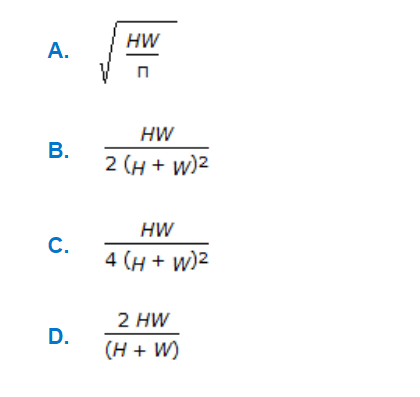a.

A

b.

B

c.

C

d.

D

Q32.
A pipe has a porous section of length L as shown in the figure. Velocity at the start of this section of V₀. If fluid leaks into the pipe through the porous section at a volumetric rate per unit area q(x/L)², what will be axial velocity in the pipe at any x ? Assume incompressible one dimensional flow i.e., no gradients in the radial direction.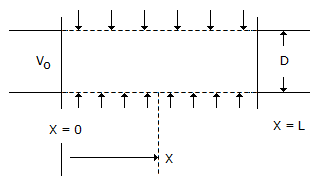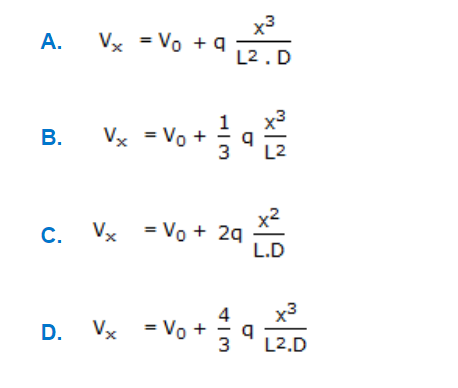a.

A

b.

B

c.

C

d.

D

Q33.
A free jet of water of cross-sectional area 0.01 m² and a velocity of 20 m/s strikes a plate and then flows in a plane parallel to the plate as shown in the figure below. The horizontal component of the force on the support is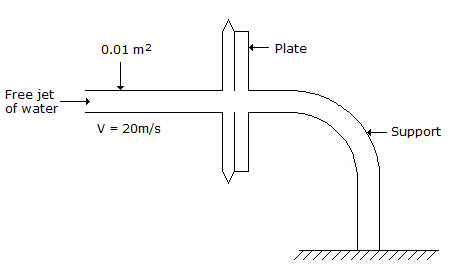Q34.
Pressure drop for laminar fluid flow through a circular pipe is given by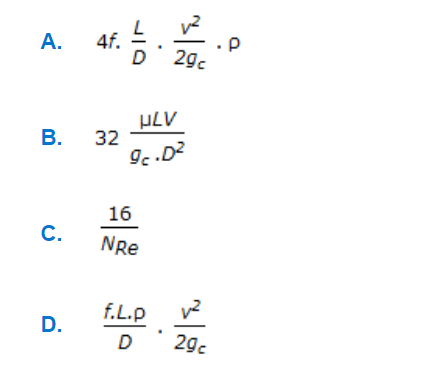a.

A

b.

B

c.

C

d.

D

Q35.
Drag co-efficient for motion of spherical particles in a stationary fluid in the stoke's law range is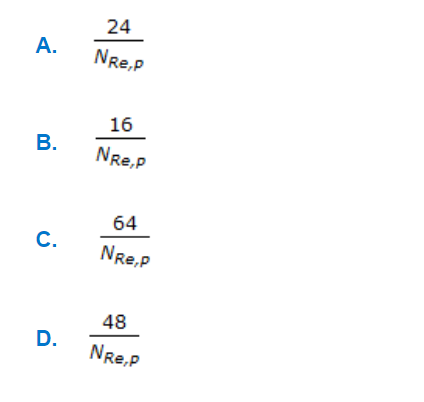a.

A

b.

B

c.

C

d.

D

Q36.
Hydraulic mean radius for flow through packed bed of spherical particle of size, 'Dₚ', with porosity 'ε' is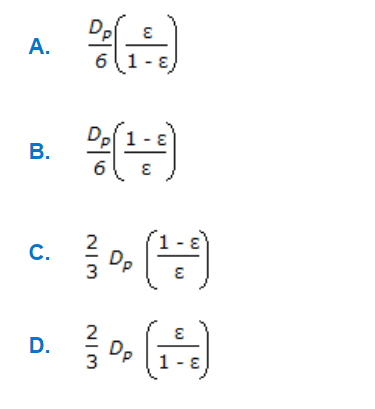a.

A

b.

B

c.

C

d.

D

Q37.
A conical tank with a bottom opening of cross-sectional area A is filled with water and is mounted on supports as shown in the figure. What is the force F with which plate X must be pushed up to prevent water from leaking ? Assume that the density of air is negligible as compared to the density of water ρL.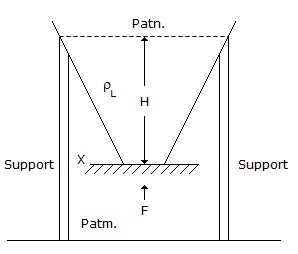Q38.
The equivalent diameter for pressure drop calculation for a duct of square cross-section is given by(where, x = each side of the square duct)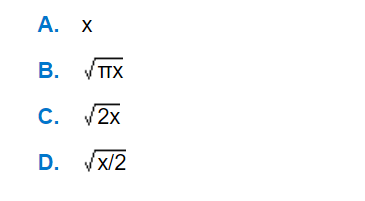a.

A

b.

B

c.

C

d.

D

Q39.
Each term of the Bernoulli's equation written in the following form, represents the total energy per unit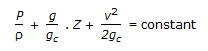Q40.
For one dimensional flow of an incompressible fluid in unsteady state in x-direction, the continuity equation is given by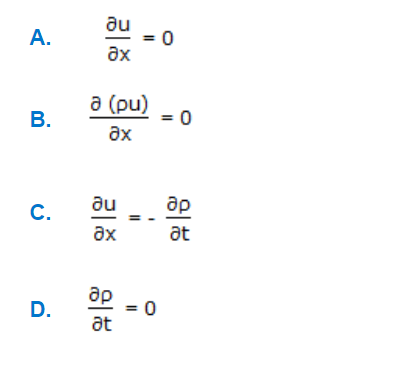a.

A

b.

B

c.

C

d.

D